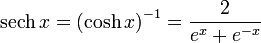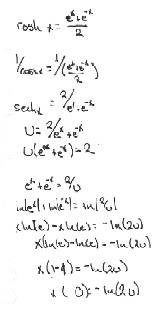# Hyperbolic Functions_Defining Sech^-1 x

Beamsbox
I have a problem that deals with the inverse sech function. sech-1 x

Trouble is I don't know how to define this in terms of ex

Such as:How would I represent the function sech^-1 x, by definition?

## Answers and Replies

Homework Helper
By the property that it's the inverse of sech of x, of course, at least where sech x is bijective.

So

\sech \left(\sech^{-1} x\right) = x

Beamsbox
\sech \left(\sech^{-1} x\right) = x

Sorry, I'm not quite sure what you've written here...

Perhaps it's the definition of inverse, that I'm not 'seeing'...

Say, for x/y, the inverse would be xy?

For, x+y/z, the inverse would be (z(y-x))...? Exactly which calculations do you 'invert', all of them?

I'm going to look more into this, @:
http://en.wikipedia.org/wiki/Inverse_function

Last edited:
Homework Helper
The tex code didn't parse correctly, or I haven't written the right code for the sech function.

So the approach to find the inverse of y=f(x) is to express x=g(y). Can you do that ?

Beamsbox
I know how to do that for a simple function like y=(x+2)/3, but I'm not sure with this one, how to go about it... here's an attempt, any insights would be nice. ;)I thik that once I have a definition for sech-1 x, that I can move forward on my own...

Beamsbox
make x = sech y; hence: y = sech-1 x

Define sech in terms of ey, then solve for y.

No u-substitution necessary, but the quadratic equation comes into play.

Thanks for your help, bigubau.